• matlab课程设计方案matlab的rc串联电路频率响应特性分析.docx
• 课程设计任务书 学生姓名 专业班级 指导教师 工作单位 题 目 Matlab应用课程设计 基于Matlab的RC串联电路频率响应特性分析 初始条件 Matlab6.5以上版本软件 先修课程电路原理等 要求完成的主要任务包括课程设计工作...
• Matlab课程设计－基于Matlab的RC串联电路频率响应特性分析
• 压缩包中包含了一阶RC电路仿真的Matlab代码以及对应课程报告。通过时域和频域两种方法对同一电路进行仿真验证，同时保证误差可控，对于刚接触电路新手具有一定参考价值
• 今天刚做了个matlab的RC电路实验仿真。只是自己留作纪念， 1.首先搭建RC电路，如图所示： 2、运行仿真后，得到波形 3.构建传递函数 4.运行仿真后，得到波形 仿真结果与理论结果一致。 注意事项： 1.需加入power...
今天刚做了个matlab的RC电路实验仿真。只是自己留作纪念，
1.首先搭建RC电路，如图所示：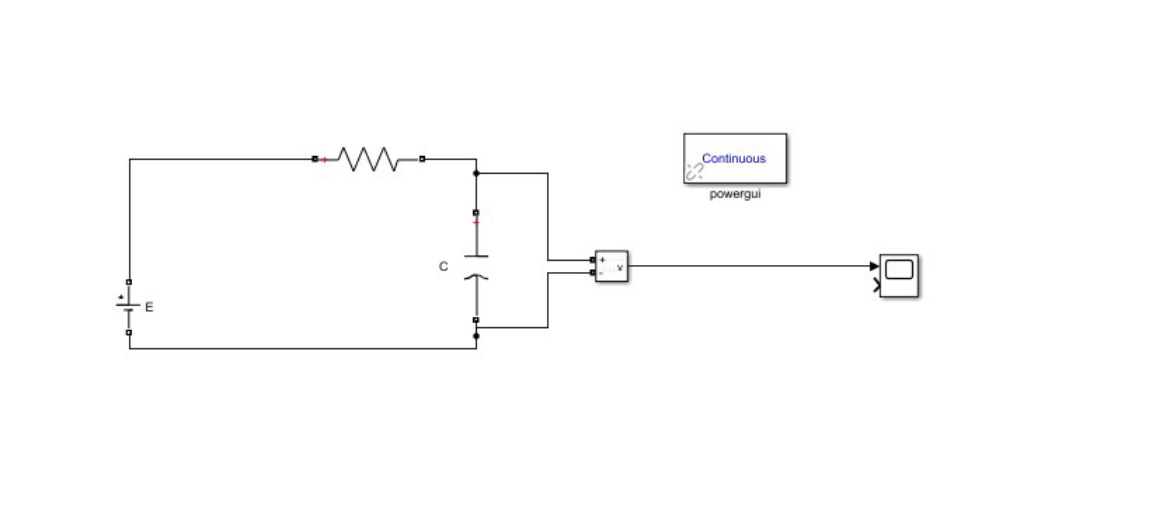2、运行仿真后，得到波形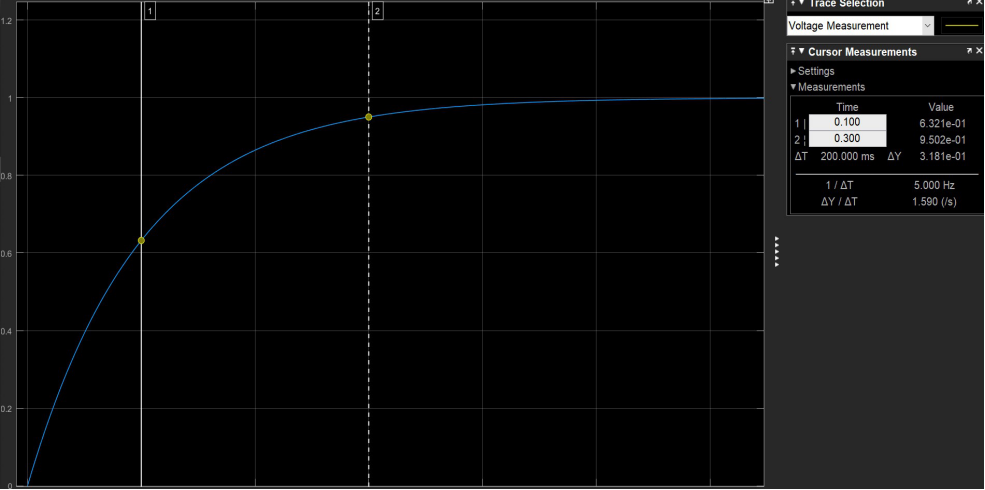3.构建传递函数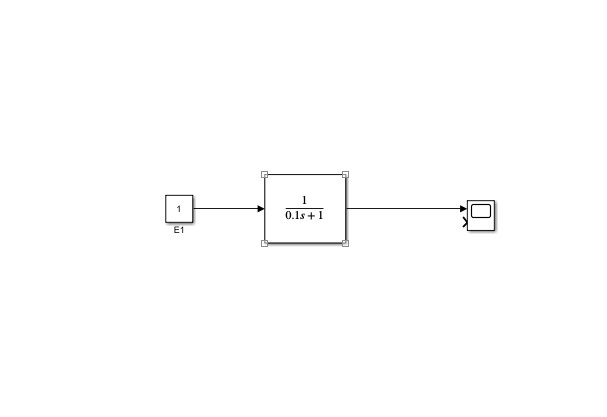4.运行仿真后，得到波形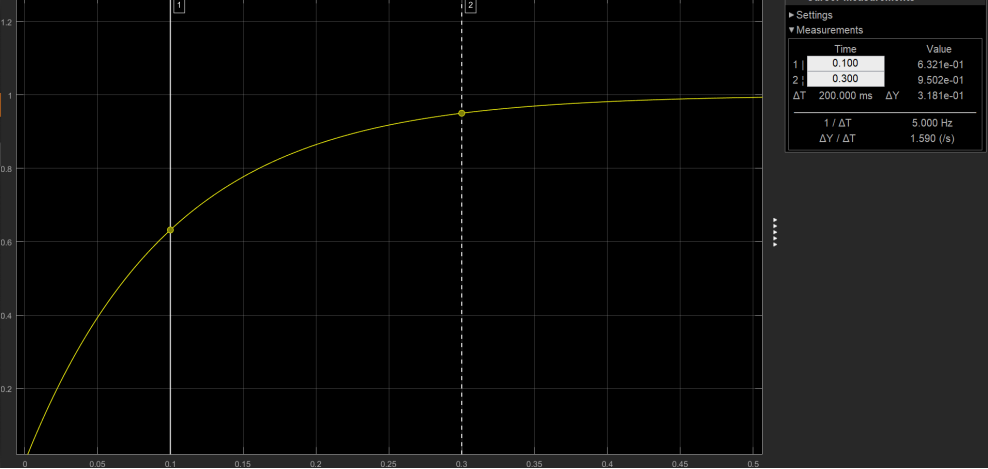仿真结果与理论结果一致。
注意事项：
1.需加入powergui模块插件
2.所有得模块选择必须在同一系统下，否则不能相互作用
3.电容两端初始电压须调为0
4.若调仿真步长，右键再点击Configuration Parameters选项，进入Slove Detail设置


展开全文simulink
• ## 【现代密码学】用MATLAB实现RC4算法

千次阅读 热门讨论 2017-12-02 16:44:01
MATLAB实现RC4算法。我写这篇博客目的主要是因为在网上很少有关于RC4算法的MATLAB实现，用java、c语言等实现很多。因此我就想用MATLAB来实现一下，以弥补这空白。
【现代密码学】用MATLAB实现RC4算法

这篇博客是我的第一篇博客，写得不太好，请多多原谅。

我写这篇博客的目的主要是因为在网上很少有关于RC4算法的MATLAB实现，用java、c语言等实现的很多。因此我就想用MATLAB来实现一下，以弥补这空白。

RC4算法我在这里就不介绍，有兴趣的点RC4算法，这个链接去了解它的原理吧，链接的那篇博客说的挺好的。

这里RC4算法，我写了5个m文件函数，分别如下：

rc4.m
—这个文件是主函数文件，通过调用一下四个函数m文件来实验RC4算法
rc4_decode.m
—这个文件是解密函数
rc4_encode.m
—这个文件是加密函数
ksa.m
—这个文件是密钥调度算法（KSA,Key-Scheduling Algorithm）函数，用来置乱S盒的初始排列
prga.m
—这个文件是伪随机生成算法（PRGA,Pseudo Random-Generation Algorithm）函数，用来输出随机序列并修改S的当前排列顺序。

MATLAB代码实现

rc4.m函数文件

function ciphertext=RC4Code(plaintext,key,flag)
%RC4加密解密算法，输出密文
%plaintext 明文字符串
%key 密钥字符串
%flag 决定加密还是解密，0为解密，其他为加密
%made by Canlong Zhang
%初始化密文
ciphertext=[];
%s盒
s=[];
%获取明文长度
plaintextLength=length(plaintext);
%生成的密钥流
keySchedual=[];
%密钥调度算法
s=ksa(s,key);
%RPGA-伪随机生成算法
keySchedual=prga(s,keySchedual,plaintextLength);
%将密钥流每个字节转化为ASCII数值
keySchedualtASCII=double(keySchedual);
%如果flag不等于0，则加密，等于0，则解密
if flag~=0
%加密
ciphertext=rc4_encode(plaintext,keySchedualtASCII);
else if flag==0
ciphertext=plaintext;
%解密
rc4_decode(ciphertext,keySchedualtASCII);
end
end

rc4_decode.m函数文件

function plaintext2=rc4_decode(ciphertext,keySchedualtASCII)
%解密
%ciphertext 密文
%keySchedualtASCII 密钥流的ASCII码
%made by Canlong Zhang
%获取密文长度
ciphertextLength=length(ciphertext);
%将明文每个字节转化为ASCII数值
ciphertextASCII=double(ciphertext);
plaintext2=[];
%为密文的每个字节用密钥流加密
for i=1:ciphertextLength
%将密钥流与明文字节进行异或处理，因为matlab无法直接对字节进行异或，因此在这里对数值进行异或处理
%bitxor只能对十进制数字进行异或处理
plaintextXORASCII=bitxor(ciphertextASCII(i),keySchedualtASCII(i));
plaintext2=[plaintext2 char(plaintextXORASCII)];
end
%输出明文
disp(['The ciphertext is:',ciphertext])
disp(['The decode plaintext is:',plaintext2])

rc4_encode.m函数文件

function ciphertext=rc4_encode(plaintext,keySchedualtASCII)
%加密
%plaintext 明文
%keySchedualASCII 密钥流的ASCII码
%made by Canlong Zhang
%初始获密文
ciphertext=[];
%获取明文长度
plaintextLength=length(plaintext);
%将明文每个字节转化为ASCII数值
plaintextASCII=double(plaintext);
ciphertextASCII=1;
%为明文的每个字节用密钥流加密
for i=1:plaintextLength
%将密钥流与明文字节进行异或处理，因为matlab无法直接对字节进行异或，因此在这里对数值进行异或处理
%bitxor只能对十进制数字进行异或处理
ciphertextXORASCII=bitxor(plaintextASCII(i),keySchedualtASCII(i));
ciphertext=[ciphertext char(ciphertextXORASCII)];
end
%输出密文
disp(['The plaintext is:',plaintext])
disp(['The encode ciphertext is:',ciphertext])

ksa.m函数文件

function s=ksa(s,key)
%ksa-密钥调度算法-利用key来对s盒做一下置换，也就是对s和重新排序
%s 输入的s盒
%key 密钥字符串
%made by Canlong Zhang
%初始化s盒
for i=1:256
s(i)=i-1;
end
j=1;
%将密钥字符串转化为ascii码数组
keyASCII=double(key);
%获取密钥的长度+1
keyLength=length(key)+1;
for i=1:256
%i对密钥长度+1取余数
modIToKeyLength=mod(i,keyLength);
if modIToKeyLength==0
modIToKeyLength=length(key);
end
j=(j+s(i)+key(modIToKeyLength));
j=mod(j,256);
if j==0
j=256;
end
%将s盒中的角标为i和j交换
temp=s(j);
s(j)=s(i);
s(i)=temp;
end
s;

prga.m函数文件

function keySchedual=prga(s,keySchedual,plaintextLength)
%PRGA(Pseudo Random-Generation Algorithm)伪随机生成算法，用来输入随机序列并修改s的党旗排列顺序
%s s盒
%keySchedual 密钥流
%plaintextLength 明文长度
%made by Canlong Zhang
i=1;
j=1;
for k=1:plaintextLength
i=mod(i+1,256);
j=mod(j+s(i),256);
%置换s（i）和s（j）
temp=s(j);
s(j)=s(i);
s(i)=temp;
%生成密钥流数组
keySchedualIndex=mod(s(i)+s(j),256);
%因为matlab的数组的索引是从1开始，因此，在index=0时，把它赋值为256
if keySchedualIndex==0
keySchedualIndex=256;
end
keySchedual=[keySchedual char(s(keySchedualIndex))];
end
keySchedual;

加密解密结果

rc4第三个参数为决定是加密还是解密，如果为0，则是解密，否则是加密。

加密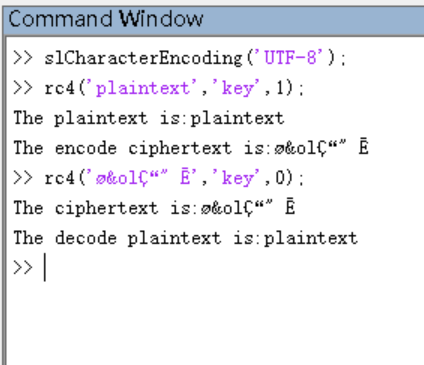解密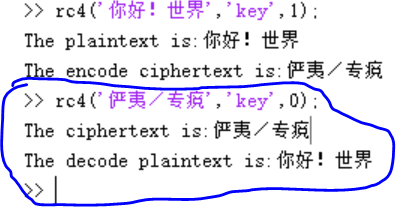一些问题

编码问题

在运行程序之前要在MATLAB软件中输入：

slCharacterEncoding('UTF-8');


来设置编码为UTF-8，因为MATLAB默认的编码为ANSI，不然会出现加密或解密乱码，从而得不到正确的明文或密文，也不能用来加密或解密中文字符了。

注意： 设定编码为UTF-8，这个很重要我曾经由于没有写设置这个编码格式，所以总是加密出来的密文显示问号，而且加密不了中文，这个问题困惑了几乎一个学期，后来偶然看到有些人的程序是有这个设置，因此我也设置了这个，最终我的程序才能运行，而且才能加密中文。

运行程序的时候要放在英文目录下运行。
不知道为什么什么在程序运行加密解密后，再设置编码输出的中文才不会乱。但是如果在运行加密解密之前设置了编码再运行程序，这是后输出中文就会乱，因此为了不乱码，我把输出的全部换成英文。

原来输出的代码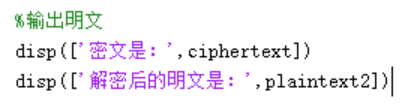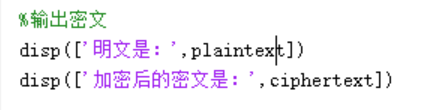没有解决的问题

不知道为什么在输出有中文的时候，第一次运行matlab，先运行后，编码，输出中文就没有问题：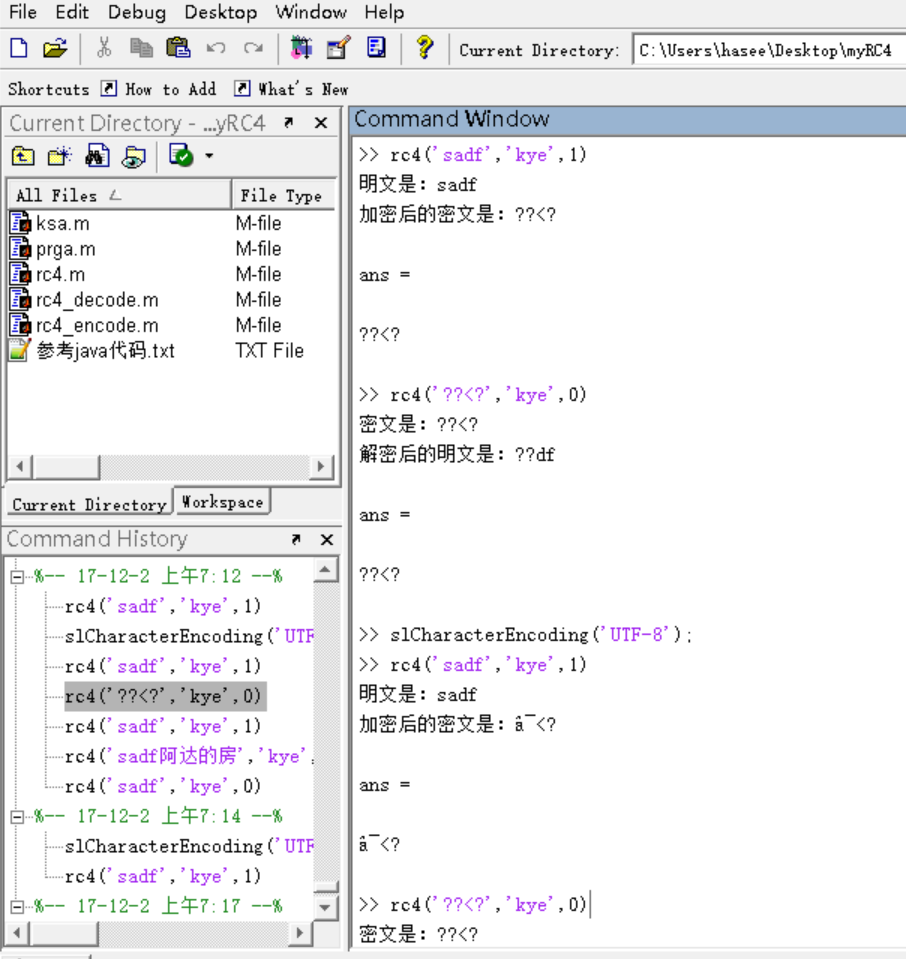但是，先关闭MATLAB后，第二次运行MATLAB，先编码后运行，输出中文就有问题：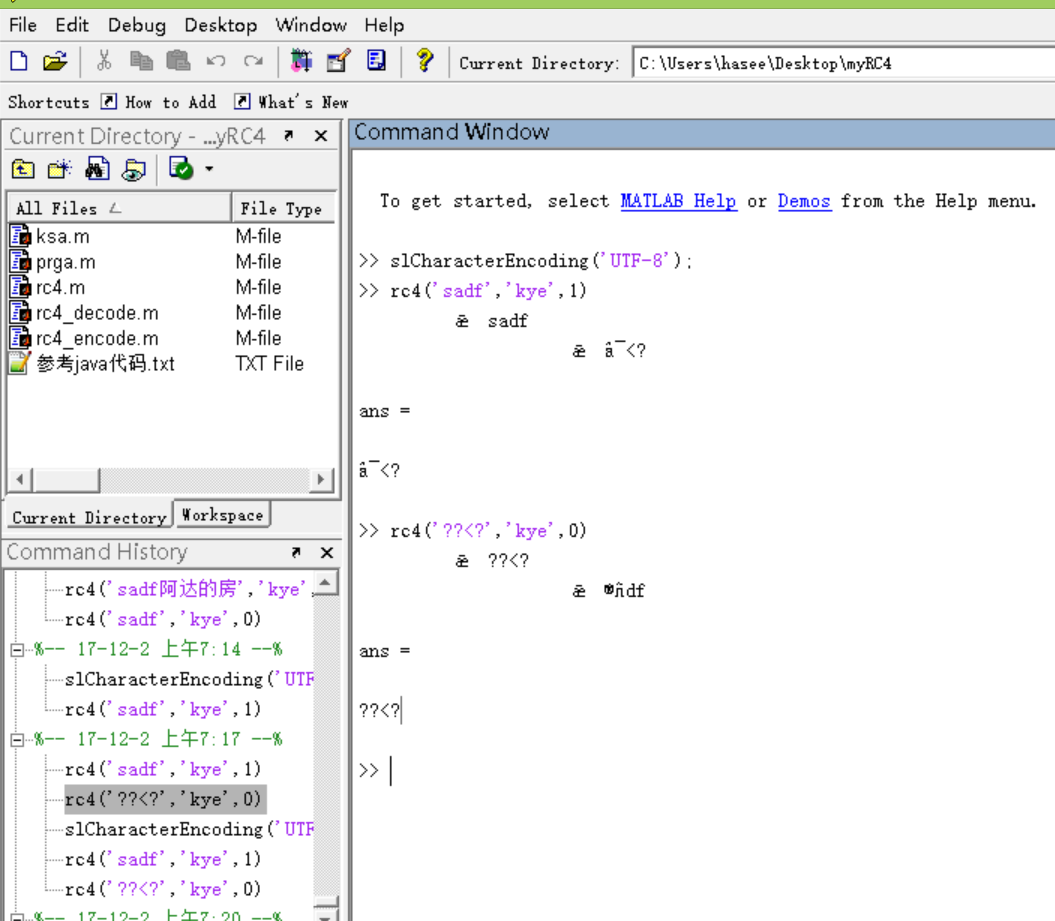展开全文• 2 2 题目用 MATLAB 对 RCRL 电路进行分 析 摘要 MATLAB 是美国 Mathworks 公司开发大型软件包是 MATrix LABoratory 缩略语目前MATLAB 广泛应用于线性代数高等数学物理 电路分析信号与系统数字信号处理自动控制等...
• ## MATLAB实现一阶RC滤波器

千次阅读 多人点赞 2019-05-31 19:55:38
MATLAB仿真分别实现一阶RC低通和高通滤波器，输入信号为正弦信号或者方波信号。 注意截止频率为f = 1/(2*pi*R*C) 低通滤波器下所示： %功能：一阶RC低通滤波器仿真 %说明： %1、分析了一阶RC滤波器幅值衰减...
MATLAB仿真分别实现一阶RC低通和高通滤波器，输入信号为正弦信号或者方波信号。

注意截止频率为f = 1/(2*pi*R*C)

低通滤波器下所示：

%功能：一阶RC低通滤波器仿真
%说明：
%1、分析了一阶RC滤波器的幅值衰减特性和相移特性
%2、分析了一阶RC滤波器的频域特性
%3、使用lsim对系统进行仿真
%4、使用FFT对原始输入信号和滤波器输出信号进行分析
%传递函数：sys=1/(1+sRC)
%==========================================================================
clc
clear
close all
A=1;       % A 幅度值
fs=5000000; % fs 采样率//最后转化为频域的最远距离最大频率//采样的频率
F=10000;   % F 频率,小于采样率的一半（奈奎斯特）//信号频率

N=5000;   % N 采样个数//取了多少个点//
%采样频率与采样个数没有什么必然关系，采样个数乘上采样频率的倒数（时间间隔）即为总的时间长度
dt=1/fs;    %时间间隔//决定了时间点与点之间的距离，当然随便指定一个数也行，只不过使信号的时域上的长度不同
t=0:dt:(N-1)*dt;    %时间向量
F1=1.0*10^3;
F2=5.0*10^3;
F3=8.0*10^3;

F4=25*10^3;
F5=45*10^3;
F6=65*10^3;

F7=100*10^3;
F8=300*10^3;
F9=400*10^3;
F10=15.9*1000;
%------------------------信源产生-------------------------------------------
dataSourceType=0;   %波形类型 0表示正弦波，1表示三角波，2表示方波
switch dataSourceType
case 0
%        y=A*sin(2*pi*F1*t);
%       y=A*sin(2*pi*F2*t);
%       y=A*sin(2*pi*F3*t);
%       y=A*sin(2*pi*F4*t);
%     y=A*sin(2*pi*F5*t);
%       y=A*sin(2*pi*F6*t)
%      y=A*sin(2*pi*F7*t);
%      y=A*sin(2*pi*F8*t);
%      y=A*sin(2*pi*F9*t);
y=A*sin(2*pi*F10*t);

case 1
y=A*sawtooth(2*pi*F*t,0.5);   %三角波
case 2
y=A*square(2*pi*F*t,50);
%方波
otherwise
end
%--------------------画出原始输入信号的时域与频域图像----------------------
figure(1);
subplot(2,1,1);
plot(t,y);
title('信号的时域波形');%对原始图像进行时域画图
xlabel('时间/s');
ylabel('电压/v');
h=fft(y,5000);%快速傅里叶变换
h_d=abs(fftshift(h));%使频域图像中间为零
f=(-N/2:N/2-1)*fs/N;%将取得时间上的点转化为频率上的点
subplot(2,1,2);
plot(f,h_d/5000);%画原始图像频域上图
%axis([-4*10^4 4*10^4 0 1]);
title('信号的频域波形');
xlabel('频率/hz');
ylabel('电压/v');
%---------------------------滤波器部分------------------------------------
%-----------------------滤波器本身特性（波特图）----------------------------
r=10.2*10^3;%电阻阻值（ome）
c=((1200*5600)/(1200+5600))*10^(-12);%电容（f）
w=2*pi*f;%角频率
Para=r*c*1i;
for n=1:length(f)
S(n)=abs(1/(1+Para*w(n)));%滤波器对于不同频率幅值衰减系数
P(n)=angle(1/(1+Para*w(n)))*180/pi;%滤波器对于不同频率相移系数
end
figure(2);
subplot(2,1,1);
plot(f,S,'r');%幅值曲线
title('幅值衰减特性');
xlabel('频率/hz');
ylabel('增益倍数');
subplot(2,1,2);
plot(f,P,'blue');%相位曲线
title('相位特性');
xlabel('频率/hz');
ylabel('相位');
Func=tf(1,[r*c,1]);%系统的传递函数
figure(3);
bode(Func);%系统的波特图
title('幅频特性');
%--------------------信号通过滤波器----------------------------------
[yout,tout] = lsim(Func,y,t);%滤波后信号图像
%-----------------------时域图像------------------------------------
figure(4);
subplot(2,1,1);
plot(t,y);
title('原始信号');
xlabel('时间/s');
ylabel('电压/v');
subplot(2,1,2);
plot(tout,yout);
title('滤波后的时域波形');
xlabel('时间/s');
ylabel('电压/v');
%------------------------------频域图像---------------------------------
q=fft(yout);
q_d=abs(fftshift(q));
figure(5);
subplot(2,1,1);
plot(f,q_d);
title('滤波后的频谱');
xlabel('频率/hz');
ylabel('电压/v');
subplot(2,1,2);
plot(f,h_d);
title('输入信号的频谱');
xlabel('频率/hz');
ylabel('电压/v');
%-------------------------------功率谱------------------------------------
figure(6);
subplot(2,1,1);
youtpsd=q_d.*conj(q_d);
plot(f,youtpsd);
title('输出信号功率谱');
xlabel('频率/Hz');
ylabel('W/Hz');
subplot(2,1,2);
ypsd=h_d.*conj(h_d);
plot(f,ypsd);
title('输入信号功率谱');
xlabel('频率/Hz');
ylabel('W/Hz');
%-------------------------------自相关函数--------------------------------
figure(7)
subplot(2,1,1);
[Rx,maxlags]=xcorr(y,'unbiased');  %信号的自相关
if fs>10000  %调整时间轴单位及标签,便于观测波形
plot(maxlags/fs*1000,Rx/max(Rx));
else
plot(maxlags/fs,Rx/max(Rx));
end
title('输入信号自相关函数');
xlabel('时间/s');
ylabel('R(t)');
subplot(2,1,2);
[Rx1,maxlags1]=xcorr(yout,'unbiased');  %信号的自相关
if fs>10000  %调整时间轴单位及标签,便于观测波形
plot(maxlags1/fs*1000,Rx1/max(Rx));
else
plot(maxlags1/fs,Rx1/max(Rx));
end
title('输出信号自相关函数');
xlabel('时间/s');
ylabel('R(t)');



高通滤波器如下所示：

%功能：一阶RC滤波器仿真
%说明：
%1、分析了一阶RC滤波器的幅值衰减特性和相移特性
%2、分析了一阶RC滤波器的频域特性
%3、使用lsim对系统进行仿真
%4、使用FFT对原始输入信号和滤波器输出信号进行分析
%传递函数：sys=s/(s+1/RC)
%==========================================================================
close all;
clear all;

%%
%输入信号的产生
%输入信号产生
fs=5000000; % fs 采样率
F=10000;   % F 频率,小于采样率的一半（奈奎斯特）
A=1;       % A 幅度值
N=5000;   % N 采样个数
%采样频率与采样个数没有什么必然关系，采样个数乘上采样频率的倒数（时间间隔）即为总的时间长度
dt=1/fs;    %时间间隔
t=0:dt:(N-1)*dt;    %时间向量
freqPixel=fs/N;     %频率分辨率，即点与点之间频率单位
dataSourceType=2;   %波形类型 0表示正弦波，1表示双音正弦波，2表示方波
F1 = 20e+3;
F2 = 5e+3;
F3 = 25e+3;
switch dataSourceType
case 0
y=A*sin(2*pi*F1*t);	%频率为20khz正弦波
case 1
y=A*(sin(2*pi*F2*t)+sin(2*pi*F3*t) ); %频率为25khz和5khz双音正弦波
case 2
y=A*square(2*pi*F2*t,50);%频率为5KHz的方波
otherwise
end
% Tlabel='时间/s';
% if fs>10000  %调整时间轴单位及标签,便于观测波形
%     t=t*1000;
%     Tlabel='时间/ms';
% end
figure(1);
subplot(2,1,1);
plot(t,y);
title('信号的时域波形');%对原始图像进行时域画图
xlabel('时间/s');
ylabel('电压/v');
h=fft(y,5000);%快速傅里叶变换
h_d=abs(fftshift(h));%使频域图像中间为零
f=(-N/2:N/2-1)*fs/N;%将取得时间上的点转化为频率上的点
subplot(2,1,2);
plot(f,h_d/5000);%画原始图像频域上图
title('信号的频域波形');
xlabel('频率/hz');
ylabel('电压/v');
% axis([-5e+5 5e+5 0 0.6]);
axis([-5e+5 5e+5 0 0.8]);
%% 滤波器的设置
% f=1:1:F;%频率序列
w=2*pi*f;
R=1.0010e+05;%电阻值
C=100e-12;%电容值
Fc=1/(2*pi*R*C);%截止频率
Para=R*C*1i;
for n=1:length(f)
A(n)=abs(1/(1+(1/(Para*w(n)))));%幅值衰减系数
P(n)=angle(1/(1+(1/(Para*w(n))))) * 180 / pi;%相移系数
end
figure(2);
subplot(2,1,1);
plot(f,A,'r');%幅值曲线
axis([0 3e+5 0 1]);
title('幅值衰减特性');
subplot(2,1,2);
plot(f,P,'blue');%相位曲线
axis([0 3e+5 0 100]);
title('相位特性');
Func=tf([1 0],[1 1/(R*C)]);%系统的传递函数
figure(3);
bode(Func);%系统的波特图
title('幅频特性');

%% 输出信号
[yout,tout] = lsim(Func,y,t);%滤波后信号图像
%-----------------------时域图像------------------------------------
figure(4);
subplot(2,1,1);
plot(t,y);
title('原始信号');
xlabel('时间/s');
ylabel('电压/v');
subplot(2,1,2);
plot(tout,yout);
title('滤波后的时域波形');
xlabel('时间/s');
ylabel('电压/v');
%------------------------------频域图像---------------------------------
q=fft(yout);
q_d=abs(fftshift(q));
figure(5);
subplot(2,1,1);
plot(f,h_d/5000);
title('输入信号的频谱');
xlabel('频率/hz');
ylabel('电压/v');
% axis([-5e+5 5e+5 0 0.6]);
axis([-5e+5 5e+5 0 0.8]);
subplot(2,1,2);
plot(f,q_d/5000);
title('滤波后的频谱');
xlabel('频率/hz');
ylabel('电压/v');
% axis([-5e+5 5e+5 0 0.6]);
axis([-5e+5 5e+5 0 0.8]);
%-------------------------------功率谱------------------------------------
figure(6);
subplot(2,1,1);
ypsd=h_d.*conj(h_d);
plot(f,ypsd);
title('输入信号功率谱');
xlabel('频率/Hz');
ylabel('W/Hz');
% axis([-5e+5 5e+5 0 0.6]);
axis([-5e+5 5e+5 0 12e+6]);
subplot(2,1,2);
youtpsd=q_d.*conj(q_d);
plot(f,youtpsd);
title('输出信号功率谱');
xlabel('频率/Hz');
ylabel('W/Hz');
% axis([-5e+5 5e+5 0 0.6]);
axis([-5e+5 5e+5 0 5e+6]);
%-------------------------------自相关函数--------------------------------
figure(7)
subplot(2,1,1);
[Rx,maxlags]=xcorr(y,'unbiased');  %信号的自相关
if fs>10000  %调整时间轴单位及标签,便于观测波形
plot(maxlags/fs*1000,Rx/max(Rx));
else
plot(maxlags/fs,Rx/max(Rx));
end
title('输入信号自相关函数');
xlabel('时间/s');
ylabel('R(t)');
subplot(2,1,2);
[Rx1,maxlags1]=xcorr(yout,'unbiased');  %信号的自相关
if fs>10000  %调整时间轴单位及标签,便于观测波形
plot(maxlags1/fs*1000,Rx1/max(Rx));
else
plot(maxlags1/fs,Rx1/max(Rx));
end
title('输出信号自相关函数');
xlabel('时间/s');
ylabel('R(t)');

注意传递函数tf的用法。

tf([1 1],[1 1 1])表示响应函数(s+1)/(s^2 + s + 1)

展开全文• %1、分析了一阶RC滤波器幅值衰减特性和相移特性 %2、分析了一阶RC滤波器频域特性 %3、使用lsim对系统进行仿真 %传递函数：sys=1/(1+sRC) %===============================================================...

%功能：一阶RC滤波器仿真
%说明：
%1、分析了一阶RC滤波器的幅值衰减特性和相移特性
%2、分析了一阶RC滤波器的频域特性
%3、使用lsim对系统进行仿真
%4、使用FFT对原始输入信号和滤波器输出信号进行分析
%传递函数：sys=1/(1+sRC)
%==========================================================================
close all;
clear all;
f=1:1:5000;%频率序列
w=2*pi*f;
R=2200;%电阻值
C=0.47e-6;%电容值
for m=1:length(f)
Zc(m)=1/(2*pi*w(m)*C);%电容阻抗
end
plot(f,Zc);
title('电容阻抗');
Fc=1/(2*pi*R*C);%截止频率
Para=R*C*i;
for n=1:length(f)
A(n)=abs(1/(1+Para*w(n)));%幅值衰减系数
P(n)=angle(1/(1+Para*w(n))) * 180 / pi;%相移系数
end
figure;
subplot(2,1,1);
plot(f,A,'r');%幅值曲线
title('幅值衰减特性');
subplot(2,1,2);
plot(f,P,'blue');%相位曲线
title('相位特性');
%频域分析
Func=tf(1,[R*C,1]);%系统的传递函数
figure;
subplot(2,1,1);
bode(Func);%系统的伯德图
title('伯德图');
subplot(2,1,2);
nyquist(Func);%系统的奈氏图
title('奈氏图');
%使用lsim进行信号仿真并使用FFT进行分析
figure;
F1=0.1;
F2=100;
F3=1000;
F4=2000;
F5=300;
%
Fs=10000;%采样率
N=20000;%采样点数
n=0:N-1;
t=n/Fs;%时间序列
f=n*Fs/N;%频率序列
%
SigIn=sin(2*pi*F1*t)+5*sin(2*pi*F2*t)+10*sin(2*pi*F3*t)+30*sin(2*pi*F4*t)+2*sin(2*pi*F5*t);%原始信号
[SigOut,Tr] = lsim(Func,SigIn,t);%滤波后信号图像
subplot(2,1,1);
plot(t,SigIn);
title('原始信号');
subplot(2,1,2);
plot(Tr,SigOut);
title('滤波后的信号');
%傅里叶变换分析滤波前的信号
sigInFFT = fft(SigIn,N);%FFT变换
%幅值特性
sigInAmp = abs(sigInFFT) * 2 / N;
sigInAmp(1) = sigInAmp(1) / 2;
%相位特性
sigInPhase = angle(sigInFFT) * 180 / pi;
figure;
subplot(2,1,1);
plot(f,sigInAmp);
title('原始信号幅值特性');
subplot(2,1,2);
plot(f,sigInPhase);
title('原始信号相位特性');
%傅里叶变换分析滤波后的信号
sigOutFFT = fft(SigOut,N);%FFT变换
%幅值特性
sigOutAmp = abs(sigOutFFT) * 2 / N;
sigOutAmp(1) = sigOutAmp(1) / 2;
%相位特性
sigOutPhase = angle(sigOutFFT) * 180 / pi;
figure;
subplot(2,1,1);
plot(f,sigOutAmp);
title('输出信号幅值特性');
subplot(2,1,2);
plot(f,sigOutPhase);
title('输出信号相位特性');


展开全文• 本人刚接触到matlab的实验仿真，由于对软件并不是特别了解，中途踩了很多坑。我希望把我仅有的一些经验分享给大家
• 在网上搜了很长时间都没找到二阶无源RC滤波参数的MATLAB仿真，没办法，自己编了。二阶RC滤波回路分析可采用拉式变换法进行比较简单，令1/CS代替C即得容抗，通过分压电路分析即得二阶RC滤波电路传递函数，另wj...工具 c
• MATLAB求解一阶RC电路和二阶RLC电路理论知识：交互界面介绍一阶RC全相应Multisim仿真：仿真结果为：二阶RLC电路Multisim仿真：仿真结果为：实现代码： 理论知识： 一阶RC电路全相应：根据节点电压法求出戴维宁等效...
• 因为最近有做一些RC滤波电路东西，算是将电路知识复习了下，也算是做一些整理，并且将推倒结果在matlab上进行仿真实验，以验证其正确性，下面给出具体过程 四阶RC低通滤波电路 四阶RC低通滤波电路如下图所示...
• (首先声明我使用环境为matlab2008a,matlab编译器版本为Compiler 4.8,VC环境为VC++6.0) 参照网上方法将m文件编译为动态链接库，然后在VC测试程序里使用该动态链接库。在这个过程中遇到两个问题，下面是这俩个...
• 这是选自国外杂志“CircuitCellar”上一篇技术文章，介绍如何用Matlab和Arduino制作一个4自由度机械臂。这是文章第一部分，第二部分会在后续文章中再做介绍。Using MATLAB and ArduinoWhen it comes to ...
• 基于实际模型RC电路 预测和最优控制 通过usb接口于matlab联接 端口驱动
• 文章目录预备知识 预备知识 一阶RC低通滤波器可以滤除频率高于截止频率信号，类似还有高通滤波器，带通滤波器，带阻滤波器，低通滤波器 C simulink
• 第一节：滤波器基本...按电路组成分：LC无源、RC无源、由特殊元件构成无源滤波器、RC有源滤波器。按传递函数微分方程阶数分：一阶、二阶、高阶。二、模拟滤波器传递函数与频率特性模拟滤波器传递函数模拟...
• 一阶RC高通滤波器的matlab仿真和C语言实现，有图有代码，欢迎拍砖数字滤波器 simulink
• %RC滤波器截止频率 f=1; %方波频率1Hz omega=2*pi*f; %基频角频率w %计算 t=-1:0.001:1 %坐标轴从-1到1，分辨率为0.001 n=[1:2:201]; %表示201谐波数叠加 单行矩阵：1,3,5,7,9...201 An=4./(pi*n); %方波...
• 滤波器分类无源滤波器一阶RC无源低通滤波器：一阶RC无源高通滤波器：无源滤波器总结：两者比较：无源滤波缺点：1、带负载能力差2、无放大作用3、特性不理想，边沿不陡。 -20dB。有源滤波电路 一阶有源滤波电路一阶低...
• 本m文件基于matlab使用firpm函数计算出滤波器系数，从而完成了使用FIR滤波器拟合一阶RC电路功能。这种思想可以扩展到其他传输函数上。
• 文章：（医学三维重建）MATLAB体绘制算法：光线投射（RCdemo
• Matlab官网上也有锂电池建模视频，但我似乎只找得到年份比较老，如果有同志找到比较新资料，欢迎交流。Lithium Battery Model with Thermal Effects for System-Level Analysis - Video​ww2.mat...
• 追求电子装置便携性以及电池供电一直是一种应用需求，其可广泛应用于医疗、远程数据采集和电力监控等领域，在某些情况下，出于对空间、功率和可靠性考虑，系统供电最好1.5V单电池，然而不幸是，绝大多数线性...
• 定义低通滤波器：允许低于一定值低频信号无衰减地通过，高于一定值信号按不同程度地被衰减、阻挡。有源低通滤波器是由有源元件和一部分无源元件(电阻、电容、电感)共同组成低通滤波器。有源元件指是必须要有...
• 倍压整流电路结构优缺点分析倍压整流电路实质是电荷泵。在一些需用高电压、小电流地方，常常使用倍压整流电路。倍压整流，可以把较低交流电压，用耐压较低整流二极管和电容器，“整”出一个较高直流电压...
• 修改matlab的默认打开路径，即自己想要的工作目录，省去自己改来改去的麻烦，怎样才能设置成自己想要的目录呢，如下两种方法，本人比较喜欢第一种： 1、Matlab的快捷方式 -> 属性 -> 起始位置 -> 更改为所希望的...
• 下面介绍常用滤波电路常见低通滤波电路L 一阶滤波C 一阶滤波CL 二阶滤波RC 二阶滤波LC 二阶滤波RCR T型三阶滤波LCL T型三阶滤波CRC π三阶滤波CLC π三阶滤波开关电源 单级低通滤波回路DLC 型二阶滤波器开关电源 双......

# matlab的rcmatlab 订阅